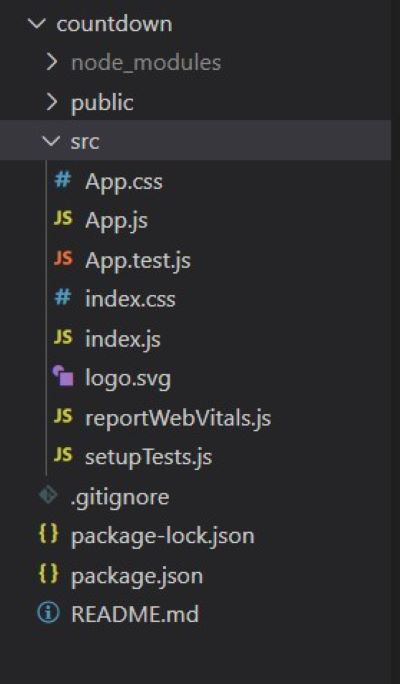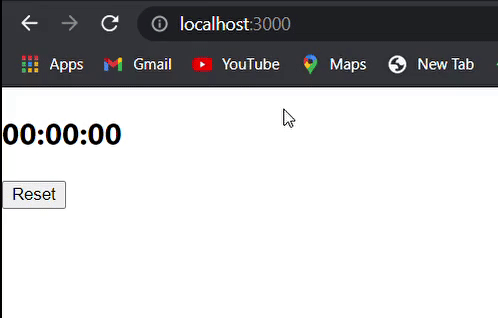Related Articles

# How to Create a Countdown Timer Using ReactJS ?

• Difficulty Level : Hard
• Last Updated : 14 Jul, 2021

In this article, we will see how to create a countdown timer using React JS. Basically, a countdown timer will show the time of the end or beginning of an offer or event. It mostly used when there is upcoming sales or big thing should we happen.

Approach:

We can use the following approach in React JS to use the Countdown timer.

• getTimeRemaining: This will compute the difference between the target timer and the current time we have. This function will check the time left from the target timer by doing calculations and return a total number of hours, minutes, and seconds.
• StartTimer: This function will start timing down from getting a total number of hours, minutes, and seconds from getTimeRemaining function.
• ClearTimer: This function is use to reset the timer, means If you restart timer it clears time remaining from the previous countdown, otherwise it starts parallel two-timing down or it may collapse each other.
• getDeadTimer: This function provides the deadline of the timer means it gives time from where you want to start the countdown. In this, you have to add time if you want to extend. We have used this in two scenarios first when the page is load and second when someone clicks reset button.

Creating React Application And Installing Module:

• Step 1: Create a React application using the following command:

`npx create-react-app foldername`
• Step 2: After creating your project folder i.e. foldername, move to it using the following command:

`cd foldername`

Project Structure: It will look like the following.Example: Now lets see how to create a countdown timer in Reactjs. The code for App.js will look like the following.

## App.js

 `import React, { useState, useRef, useEffect } from ``'react'`` ` ` ` `const App = () => {`` ` `    ``// We need ref in this, because we are dealing``    ``// with JS setInterval to keep track of it and``    ``// stop it when needed``    ``const Ref = useRef(``null``);`` ` `    ``// The state for our timer``    ``const [timer, setTimer] = useState(``'00:00:00'``);`` ` ` ` `    ``const getTimeRemaining = (e) => {``        ``const total = Date.parse(e) - Date.parse(``new` `Date());``        ``const seconds = Math.floor((total / 1000) % 60);``        ``const minutes = Math.floor((total / 1000 / 60) % 60);``        ``const hours = Math.floor((total / 1000 * 60 * 60) % 24);``        ``return` `{``            ``total, hours, minutes, seconds``        ``};``    ``}`` ` ` ` `    ``const startTimer = (e) => {``        ``let { total, hours, minutes, seconds } ``                    ``= getTimeRemaining(e);``        ``if` `(total >= 0) {`` ` `            ``// update the timer``            ``// check if less than 10 then we need to ``            ``// add '0' at the begining of the variable``            ``setTimer(``                ``(hours > 9 ? hours : ``'0'` `+ hours) + ``':'` `+``                ``(minutes > 9 ? minutes : ``'0'` `+ minutes) + ``':'``                ``+ (seconds > 9 ? seconds : ``'0'` `+ seconds)``            ``)``        ``}``    ``}`` ` ` ` `    ``const clearTimer = (e) => {`` ` `        ``// If you adjust it you should also need to``        ``// adjust the Endtime formula we are about``        ``// to code next    ``        ``setTimer(``'00:00:10'``);`` ` `        ``// If you try to remove this line the ``        ``// updating of timer Variable will be``        ``// after 1000ms or 1sec``        ``if` `(Ref.current) clearInterval(Ref.current);``        ``const id = setInterval(() => {``            ``startTimer(e);``        ``}, 1000)``        ``Ref.current = id;``    ``}`` ` `    ``const getDeadTime = () => {``        ``let deadline = ``new` `Date();`` ` `        ``// This is where you need to adjust if ``        ``// you entend to add more time``        ``deadline.setSeconds(deadline.getSeconds() + 10);``        ``return` `deadline;``    ``}`` ` `    ``// We can use useEffect so that when the component``    ``// mount the timer will start as soon as possible`` ` `    ``// We put empty array to act as componentDid``    ``// mount only``    ``useEffect(() => {``        ``clearTimer(getDeadTime());``    ``}, []);`` ` `    ``// Another way to call the clearTimer() to start``    ``// the countdown is via action event from the``    ``// button first we create function to be called``    ``// by the button``    ``const onClickReset = () => {``        ``clearTimer(getDeadTime());``    ``}`` ` `    ``return` `(``        ``
``            ``

{timer}

``            ````        ``
``    ``)``}`` ` `export ``default` `App;`

Step to Run Application: Run the application using the following command from the root directory of the project:

`npm start`

Output: Now open your browser and go to http://localhost:3000/, you will see the following output:My Personal Notes arrow_drop_up